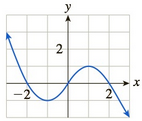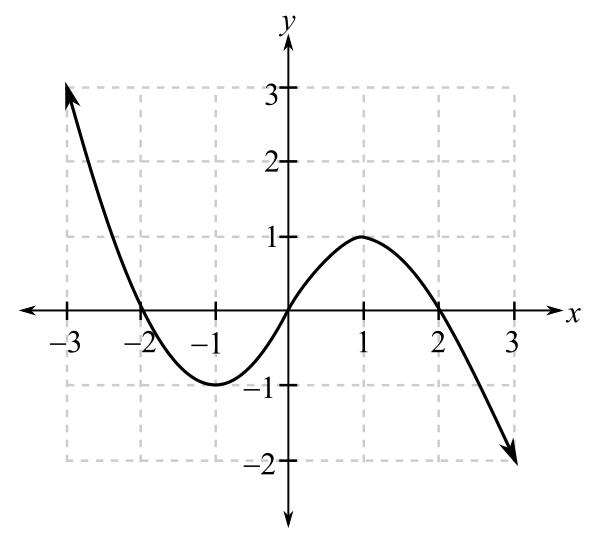Chapter 10, Problem 40REFinite Mathematics and Applied Cal...

7th Edition
Stefan Waner + 1 other
ISBN: 9781337274203

Solutions

Chapter
SectionFinite Mathematics and Applied Cal...

7th Edition
Stefan Waner + 1 other
ISBN: 9781337274203
Textbook Problem

Let f have the graph shown.Select the correct answer.a. The average rate of change of f over the interval [ 0 , 1 ] is(A) greater than f ' ( 0 ) .(B) less than f ' ( 0 ) .(C) approximately equal to f ' ( 0 ) b. The average rate of change of f over the interval [ 0 , 2 ] is(A) greater than f ' ( 1 ) .(B) less than f ' ( 1 ) .(C) approximately equal to f ' ( 1 ) .c. Over the interval [ − 2 , 0 ] theinstantaneous rate of change of f is(A) increasing.(B) decreasing.(C) neither increasing nor decreasing.d. Over the interval [ − 2 , 2 ] theinstantaneous rate of change of f is(A) increasing, then decreasing.(B) decreasing, then increasing.(C) approximately constant.e. When x = 0 , f ( x ) is(A) Approximate 0 and increasing at a rate of about 1.5 units per unit of x.(B) approximately 0 and increasing at a rate of about 1.5 unit per unit of x.(C) approximately 1.5 and neither increasing nor decreasing.(D) approximately 0 and neither increasing nor decreasing.

(a)

To determine

The average rate of change of function f in interval [0,1] from the graph and choose the correct options.

The options are:

A) Greater than f(0)

B) Less than f(0)

C) Approximately equal to f(0)

The graph provided is:Explanation

Given information:

The options are:

A) Greater than f(0)

B) Less than f(0)

C) Approximately equal to f(0)

The provided graph is:

When function is increasing or rising the slope of the function is positive and greatest where the curve is more slanted whereas when the function is decreasing or falling its slope is negative and it has the least value where the curve is the steepest.

The derivative at x=0 or f(0) is the slope of tangent at that point

(b)

To determine

The average rate of change of function f in interval [0,2] from the graph and choose the correct options.

The options are:

A) Greater than f(1)

B) Less than f(1)

C) Approximately equal to f(1)

The graph provided is:(c)

To determine

The instantaneous rate of change of function f in interval [2,0] from the graph and choose the correct options.

The options are:

A) Increasing

B) Decreasing

C) Neither increasing nor decreasing

The graph provided is:(d)

To determine

The instantaneous rate of change of function f in interval [2,2] from the graph and choose the correct options.

The options are:

A) Increasing, then decreasing

B) Decreasing, then increasing

C) Approximately constant

The graph provided is:(e)

To determine

The value of f(x) at x=0 from the graph and choose the correct options.

The options are:

A) Approximately 0 and increasing at the rate of about 1.5 units per unit of x.

B) Approximately 0 and decreasing at the rate of about 1.5 units per unit of x.

C) Approximately 1.5 and neither increasing nor decreasing.

D) Approximately 0 and neither increasing nor decreasing.

The graph provided is:Still sussing out bartleby?

Check out a sample textbook solution.

See a sample solution

The Solution to Your Study Problems

Bartleby provides explanations to thousands of textbook problems written by our experts, many with advanced degrees!

Get Started

Divide: 8x212x

Elementary Technical Mathematics

Given that for all x, a power series for is:

Study Guide for Stewart's Multivariable Calculus, 8th

True or False: If h'(x) = k(x), then k(x) is an antiderivative of h(x).

Study Guide for Stewart's Single Variable Calculus: Early Transcendentals, 8th